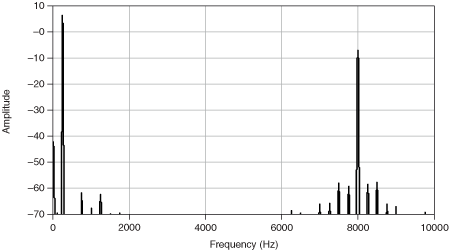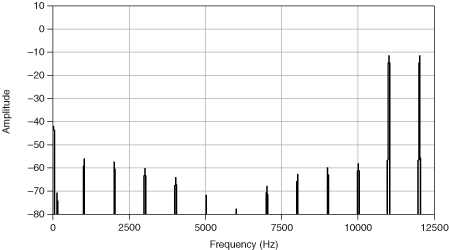# Intermodulation Distortion

## Sound and Vibration Measurement Help

Edition Date: March 2018
Part Number: 372416L-01
View Product InfoLabVIEW 2018 Sound and Vibration Toolkit HelpLabVIEW 2019 Sound and Vibration Toolkit Help

Intermodulation distortion (IMD) is the measurement of distortion due to nonlinearity in the device under test (DUT). IMD measurements use a dual-tone test signal composed of a low-frequency tone at f1 and a high-frequency tone at f2. When a dual-tone signal is the input to a nonlinear device, the device output contains intermodulation components of f1 and f2 given by m*f2 ± n*f1, where m and n are integers.

You often use IMD to measure the distortion of a DUT near the high-frequency limit of the DUT or the measurement system. You also can arrange the test so that many IMD components occur within the measurement bandwidth.

Several standard configurations for IMD measurements exist, and these configurations use one of two types of IMD test signals.

In the first type of IMD test signal, with a low-frequency modulation tone and a high-frequency carrier tone, the intermodulation components appear as sidebands around the high-frequency carrier tone, as calculated in the following equation:

f 2 ± n * f1

The following figure shows the sidebands around the 8 kHz carrier tone.In the second type of IMD test signal, with two closely spaced tones near the high-frequency limit of the measurement bandwidth, the intermodulation components appear at multiples of the difference frequency calculated by the following equation:

m * (f2 - f1)

The following figure shows a typical IMD test spectrum.The intermodulation components are found at multiples of 1,000 Hz, the difference frequency between the twin 11 kHz and 12 kHz tones.